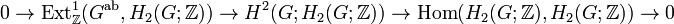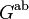# Schur covering group

## Definition

### Definition for an arbitrary group

Suppose$G$ is a group. A Schur covering group for$G$ can be defined in the following ways:

1. It is a central extension with normal subgroup (hence also central subgroup) the Schur multiplier$M(G) = H_2(G;\mathbb{Z})$ and quotient group$G$, such that under the universal coefficients theorem mapping$H^2(G;A) \to \operatorname{Hom}(H_2(G;\mathbb{Z}),A)$, with$A = H_2(G;\mathbb{Z})$, the image of its cohomology class is the identity map from$H_2(G;\mathbb{Z})$ to itself.
2. It is a stem extension$K$ with quotient group$G$ (stem extension meaning it is a central extension and the central subgroup of the extension is contained in the derived subgroup) such that the following holds: consider the commutator map homomorphism$K \wedge K \to [K,K]$. Because$G$ is a quotient by a central subgroup, this descends to a map$G \wedge G \to [K,K]$. We want the descended map to be an isomorphism.

### Alternative characterization for a finite group

For a finite group$G$, a Schur covering group (also called a Schur cover) is a stem extension where the quotient group is$G$ that is of maximum possible order among all stem extensions where the quotient group is$G$. Every finite group has a finite Schur covering group.

Here, a stem extension is a central extension where the base normal subgroup is contained in the derived subgroup of the whole group.

The base normal subgroup for a Schur covering group is always the Schur multiplier of$G$. However, not every extension whose base normal subgroup is the Schur multiplier is a stem extension.

## Uniqueness up to isoclinism

The Schur covering group is not uniquely determined up to isomorphism, but it is uniquely determined up to isoclinism. Its inner automorphism group is the quotient group of$G$ by its epicenter, and its derived subgroup is the exterior square$G \wedge G$. The stem extension assumption further tells us that all the Schur covering groups have the same order.

In group extension terms, consider the short exact sequence:$0 \to \operatorname{Ext}^1_{\mathbb{Z}}(G^{\operatorname{ab}},A) \to H^2(G;A) \to \operatorname{Hom}(H_2(G;\mathbb{Z}),A) \to 0$

Set$A = H_2(G;\mathbb{Z})$ to get:$0 \to \operatorname{Ext}^1_{\mathbb{Z}}(G^{\operatorname{ab}},H_2(G;\mathbb{Z})) \to H^2(G;H_2(G;\mathbb{Z})) \to \operatorname{Hom}(H_2(G;\mathbb{Z}),H_2(G;\mathbb{Z})) \to 0$

The Schur covering groups, as group extensions, are those elements of$H^2(G;H_2(G;\mathbb{Z}))$ that map to the identity map element of$\operatorname{Hom}(H_2(G;\mathbb{Z}),H_2(G;\mathbb{Z}))$ (which is not the zero element of that group, but rather its unit when it's viewed as a ring). These form a coset of the image of$\operatorname{Ext}^1_{\mathbb{Z}}(G^{\operatorname{ab}},H_2(G;\mathbb{Z})$. It is not necessary that all these extensions actually correspond to different isomorphism classes; there may be pseudo-congruences that give isomorphisms of the overall groups.

Case on$G$ What can we say about Schur covering groups? What is the inner automorphism group of any Schur covering group? (in general, it is the quotient of$G$ by its epicenter) What is the derived subgroup of any Schur covering group? (in general, it is the exterior square of$G$)
perfect group unique, and it is called the universal central extension nothing more specific same as the Schur covering group
capable group (any centerless group is capable, but the converse doesn't hold) nothing specific isomorphic to$G$ nothing more specific

## Cases it is unique up to isomorphism of extensions

The Schur covering group is unique if and only if the original group is a group with unique Schur covering group. A group$G$ has this property if and only if$\operatorname{Ext}^1(G^{\operatorname{ab}},M(G))$ is trivial, where$G^{\operatorname{ab}}$ is the abelianization and$M(G) = H_2(G;\mathbb{Z})$ is the Schur multiplier. This basically means that the left term in the short exact sequence vanishes, so the right map is an isomorphism, which in turn means that the inverse image of the identity map is a single unique extension.